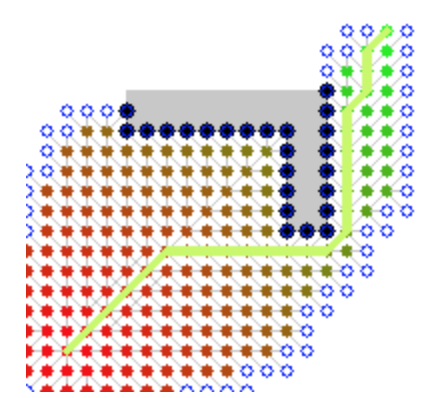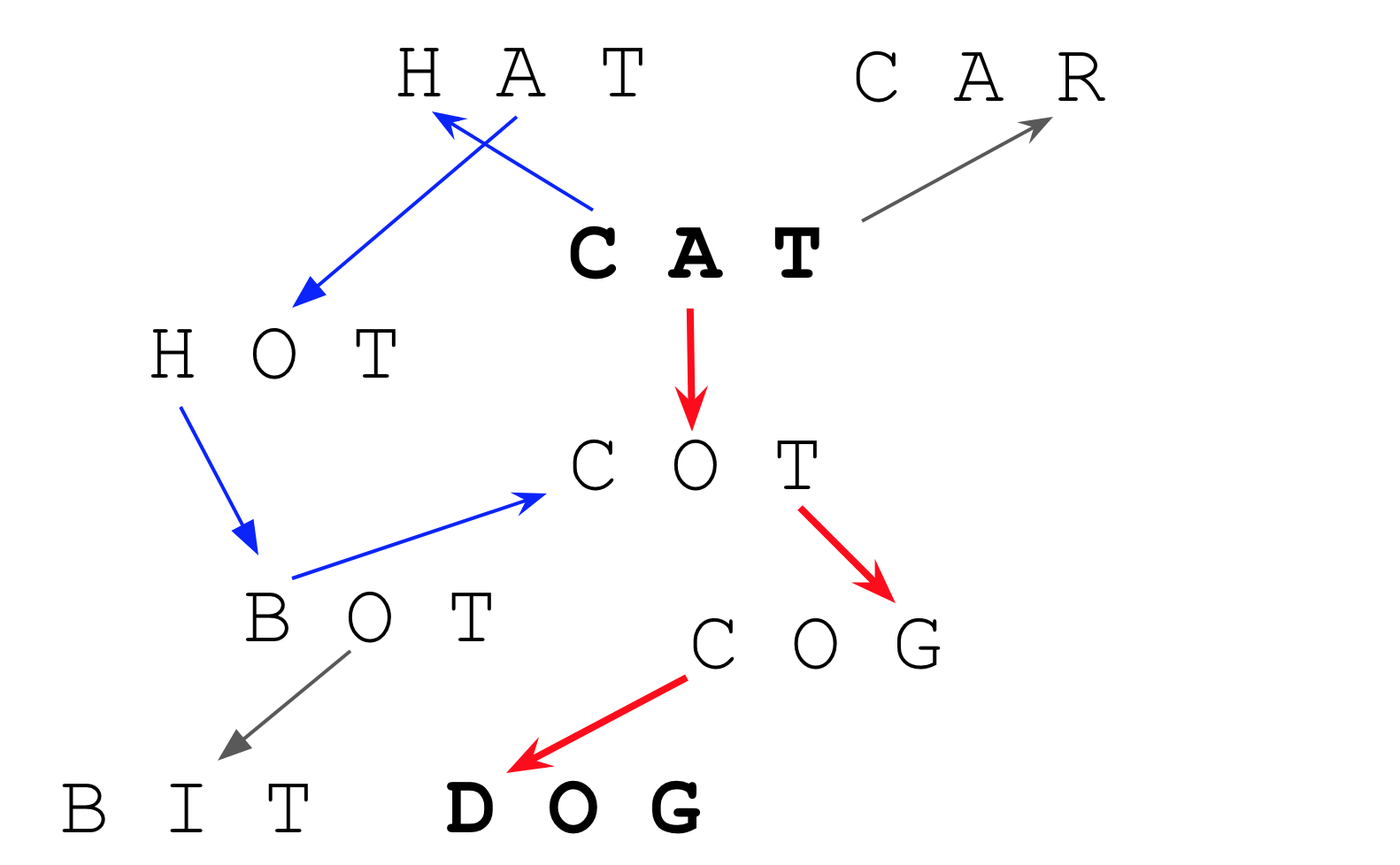# Using R and the A* Algorithm: Turning Cats into Dogs

## January 21, 2019 by Michael Chow

Recently, I’ve come across a 3 problems that were solved quickly using the A* algorithm:

1. Splitting cantonese sentences into words (e.g. 我好肚餓 -> 我 - 好 - 肚餓).
2. Comparing how similar sounding two english words are.
3. Cruising around minecraft.

Since I started on these problems using python, the python-astar package got me up and running quickly. However, when switching to R I wasn’t able to find it in any libraries, like igraph.

I’m sure it exists somewhere, but after a couple hours of searching I opted for the next best thing: writing a quick R package (machow/astar-r).

## Using A* in R

The A* algorithm (pronounced “a star”) is a search algorithm for finding the “shortest” path between two nodes in a graph.

One common use is navigating between two points in space.The image above, taken from wikipedia, shows a search for a path from the bottom left of a grid to the top right, where an obstacle (grey “¬” shape) is in the way. Solid colors are nodes on the grid that have been checked, and an optimal path (solid green line) was found to the right of the obstacle.

In the package, the astar function requires specifying functions that answer four questions for a given node:

1. Is this the goal node?
2. Who are its neighbors?
3. How far is it from a given neighbor?
4. (Approximately) how far is it from the goal?

While navigating in space is a common use case, a lot of other problems can be solved by A* as well! In order to demonstrate the A* package, I’ll use another interesting case: word ladders.

Word ladders is a game where you are given a list of words. Each word can be considered a node. How you play is defined by two pieces: a rule for what makes nodes neighbors and a goal. In this post, we’ll define those as..

1. words that differ by only one letter are neighbors (e.g. “cat” and “bat”)
2. we want to find the path from one word to another (e.g. “cat” to “dog”)

For example, the graph below shows some possible paths we could try for going from “cat” to “dog”. The red path makes it in a few steps, whereas the blue path takes a roundabout way, that meets up with the shorter path.Below, I’ll show how the A* algorithm can solve the problem of finding a path that takes the least number of steps.

First we install the astar package..

remotes::install_github('machow/astar-r')


Next, we define the nodes in our graph, along with tools to see how far they are from eachother..

library(astar)

words <- c(
'cat', 'hat', 'bat', 'bet', 'cot', 'cut', 'bed', 'bud',
'bot', 'bit', 'pat', 'sat', 'pit', 'put', 'sit', 'mit',
'bog', 'bug', 'big', 'bag', 'cog', 'pig', 'hog', 'sag',
'dig', 'dug', 'dog'
)

split <- function(s)               # breaks word into vector of letters
unlist(strsplit(s, ""))

ltr_dist <- function(s1, s2)       # number of letters that differ
sum(split(s1) != split(s2))


Then, we define four functions to answer each of the A* questions listed above. In this case, I set all nodes to be connected as neighbors, but the distance between invalid neighbors as infinite.

# A* methods ----
is_goal_reached <- function(src, dst)     # should we stop?
src == dst

neighbors <- function(node)               # find neighbors
words[words != node]

edge_distance <- function(src, dst)       # how far is node from neighbor
if (ltr_dist(src, dst) == 1) 1 else Inf

cost_estimate <- function(node, goal)     # estimate how far node is from goal
as.numeric(ltr_dist(node, goal))        # best case estimate: min swaps left


Finally, we run the astar function with the starting and ending nodes..

# Run ----
astar('cat', 'dog',
cost_estimate, edge_distance, neighbors, is_goal_reached
)

[]
 "cat"

[]
 "cot"

[]
 "cog"

[]
 "dog"


The output matches the red (shortest) path in the word ladder graph!

## The World is Full of Problems A* can Solve

While A* is often taught as a spatial pathfinding algorithm, it can solve a surprisingly diverse set of problems. More detailed explanations of the algorithm can be found on wikipedia, or this interactive article.

If you use the R astar package to solve any nifty problems, or encounter any bugs, let me know on Twitter (\@chowthedog) or on github!